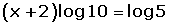Learn from home

The teachersChapters

An exponential equation is an equation in which the unknown variable is in the exponent.

To solve an exponential equation, consider:

## 1## 2## 3

The exponent rules.

a0 = 1 ·

a1 = aam · a n = am+n

am : a n = am - n

(am)n = am · n

an · b n = (a · b) n

an : b n = (a : b) n

## Solving Exponential Equations:To solve an unknown that is in the exponent, use logarithms whose base is the base of the power.Did you like the article?(1 votes, average: 5.00 out of 5)Loading...

Emma

I am passionate about travelling and currently live and work in Paris. I like to spend my time reading, gardening, running, learning languages and exploring new places.

Did you like
this resource?

Bravo!